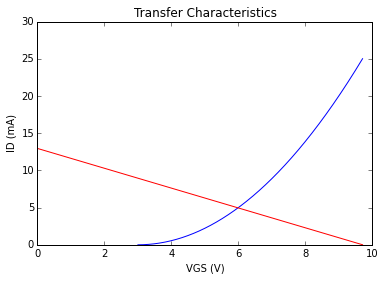# Chapter 7 : Field effect transistors - characteristics and biasing¶

## Example 7.1: Page No 346¶

In :
from numpy import arange
%matplotlib inline
from matplotlib.pyplot import plot, title, xlabel, ylabel, show

#Transfer curve

IDSS=12# # in mili-amperes
VP=-5# # in volts
# Plotting transfer curve
VGS=arange(0, VP,-0.01) # Gate source voltage in volts
# Using Shockley's equation
ID=[]
for vgs in VGS:
ID.append(IDSS*(1-vgs/VP)**2) # Drain current in mili-amperes

plot(VGS,ID)
title("Transfer Curve")
xlabel("VGS (V)")
ylabel("ID (mA)")
show()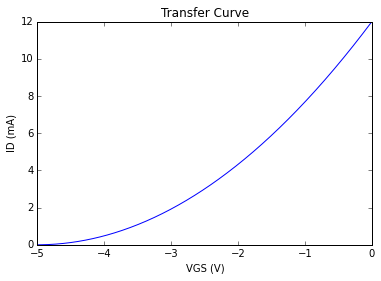## Example 7.2: Page No 352¶

In :
#              (a) Region of operation
#              (b) Region of operation
#              (c) Region of operation

VT=2# # in volts
VGS=3# # in volts
print "VGS - VT  = %0.2f V"%(VGS-VT)

print "Part (a)"
print "VDS = %0.2f V "%(0.5)
print "Since VDS < VGS - VT, therefore transistor is in ohmic region."

print "Part (b)"
print "VDS = %0.f V "%1
print "Since VDS = VGS - VT, therefore transistor is in saturation region."

print "Part (c)"
print "VDS  %0.f V "%5
print "Since VDS > VGS - VT, therefore transistor is in saturation region."

VGS - VT  = 1.00 V
Part (a)
VDS = 0.50 V
Since VDS < VGS - VT, therefore transistor is in ohmic region.
Part (b)
VDS = 1 V
Since VDS = VGS - VT, therefore transistor is in saturation region.
Part (c)
VDS  5 V
Since VDS > VGS - VT, therefore transistor is in saturation region.


## Example 7.3: Page No 359¶

In :
#IDQ, VDSQ

IDSS=12# # in mili-amperes
VP=-4# # in volts
# From Fig. 7.28
VDD=12# # in volts
RD=1.2# # in kilo-ohms
# Since IG=0
VGS=-1.5# # in volts
# Using Shockley's equation
ID=IDSS*(1-VGS/VP)**2# # Drain current in mili-amperes
VDS=VDD-ID*RD# # in volts
print "IDQ = %0.1f mA "%ID
print "VDSQ = %0.2f V "%VDS

IDQ = 4.7 mA
VDSQ = 6.38 V


## Example 7.4: Page No 361¶

In :
from sympy import symbols, solve
from numpy import arange
%matplotlib inline
from matplotlib.pyplot import plot, title, xlabel, ylabel, show

#VDSQ, IDSQ, VD, VS

IDSS=6e-3# # in amperes
VP=-6# # in volts
# From Fig. 7.31
VDD=12# # in volts
RD=2.2e3# # in ohms
RS=1.6e3# # in ohms
# Plotting transfer characteristics
VGS=arange(0, VP, -0.01) # Gate source voltage in volts
# Using Shockley's equation
ID = []
for vgs in VGS:
ID.append(IDSS*(1-vgs/VP)**2*1e3) # Drain current in mA

plot(VGS,ID)#
title("Transfer Characteristics")
xlabel("VGS (V)")
ylabel("ID (mA)")
# Plotting bias line
# From gate source circuit
ID=[]
for vgs in VGS:
ID.append(-vgs/RS*1e3) # Source current in mA
plot(VGS,ID,"RED")
show()

# Intersection of transfer characteristics with the bias curve
# Putting VGS = -ID*RS in Shockley's equation and solving, we get ID**2*RS**2 + (2*RS*VP - VP**2/IDSS)*ID + VP**2
# Solving the equation
ID = symbols('ID')
expr = ID**2*RS**2 + (2*RS*VP - VP**2/IDSS)*ID + VP**2
IDQ = solve(expr, ID) # A
# Writing the KVL for the output loop
VDSQ=VDD-IDQ*(RD+RS)# # in volts
VS=IDQ*RS# # in volts
VD=VDSQ+VS# # in volts
IDQ=IDQ*1e3# # in mili-amperes
print "VDSQ = %0.2f V "%VDSQ
print "IDQ = %0.2f mA "%IDQ
print "VD = %0.2f V "%VD
print "VS = %0.2f V "%VS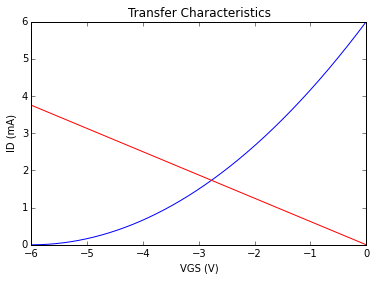VDSQ = 5.41 V
IDQ = 1.73 mA
VD = 8.19 V
VS = 2.77 V


## Example 7.5: Page No 363¶

In :
from numpy import arange
from sympy import symbols, solve
%matplotlib inline
from matplotlib.pyplot import plot, title, xlabel, ylabel, show

#Operating point

VP=-5# # in volts
IDSS=12e-3# # in amperes
# From Fig. 7.34(a)
VDD=18# # in volts
R1=400# # in kilo-ohms
R2=90# # in kilo-ohms
RD=2e3# # in ohms
RS=2e3# # in ohms
# Applying Thevnin's theorem to obtain simplified circuit in Fig. 7.34(b)
VGG=VDD*R2/(R1+R2)# # in volts
# Plotting transfer characteristics
VGS=arange(VGG, VP,-0.01) # Gate source voltage in volts
# Using Shockley's equation
ID=[]
for vgs in VGS:
ID.append(IDSS*(1-vgs/VP)**2*1e3) # Drain current in mA
plot(VGS,ID)
title("Transfer Characteristics")
xlabel("VGS (V)")
ylabel("ID (mA)")
# Plotting bias line
# From the KVL for the gate-loop
ID=[]
for vgs in VGS:
ID.append((-vgs+VGG)/RS*1e3)# # Source current in mA
plot(VGS,ID,"RED")
# Intersection of transfer curve with the bias curve
# Putting VGS = VGG-ID*RS in Shockley's equation and solving, we get
# ID**2*RS**2 + (2*RS*VP - 2*VGG*RS - VP**2/IDSS)*ID + (VGG-VP)**2
# Solving the equation
ID = symbols('ID')
expr = ID**2*RS**2 + (2*RS*VP - 2*VGG*RS - VP**2/IDSS)*ID + (VGG-VP)**2
IDQ=solve(expr) # in amperes
# Writing the KVL for the drain source loop
VDSQ=VDD-IDQ*(RD+RS)# # in volts
IDQ=IDQ*1e3# # in mili-amperes
print "VDSQ = %0.2f V "%VDSQ
print "IDQ = %0.2f mA "%IDQ

VDSQ = 6.83 V
IDQ = 2.79 mA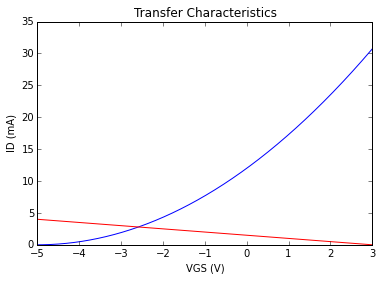## Example 7.6: Page No 365¶

In :
from numpy import arange
from sympy import symbols, solve
%matplotlib inline
from matplotlib.pyplot import plot, title, xlabel, ylabel, show

#VDSQ, IDQ

ID=6e-3# # in amperes
VGS=8# # in volts
VT=3# # in volts
# From Fig. 7.37(a)
VDD=12# # in volts
RD=2e3# # in ohms
# Plotting transfer curve
k=ID/(VGS-VT)**2# # in amperes per volt square
from numpy import arange
VGS=arange(3, VDD,0.01) # Gate source voltage in volts
ID=[]
for x in VGS:
ID.append(k*(x-VT)**2*1e3) # Drain current in mA ............ (i)
plot(VGS,ID)#
title("Transfer Curve")
xlabel("VGS (V)")
ylabel("ID (mA)")
# Plotting bias line
# From the simplified dc equivalent circuit in Fig. 7.37(b)
VGS=arange(0, VDD,0.01) # Gate source voltage in volts
ID=[]
for x in VGS:
ID.append((VDD-x)/RD*1e3)# # Source current in mA
plot(VGS,ID,"RED")
# Intersection of transfer curve with the bias curve
# Putting VGS = VDD-ID*RD in equation (i) and solving, we get ID**2*RD**2 + (2*RD*VT - 2*VDD*RD - 1/k)*ID + (VDD-VT)**2
# Solving the equation
from sympy import symbols, solve
ID = symbols('ID')
expr = ID**2*RD**2 + (2*RD*VT - 2*VDD*RD - 1/k)*ID + (VDD-VT)**2
IDQ=solve(expr, ID)#  in amperes
VGSQ=VDD-IDQ*RD# # in volts
IDQ=IDQ*1e3# # in mili-amperes
print "VDSQ = %0.2f V "%VGSQ
print "IDQ = %0.2f mA "%IDQ

VDSQ = 6.41 V
IDQ = 2.79 mA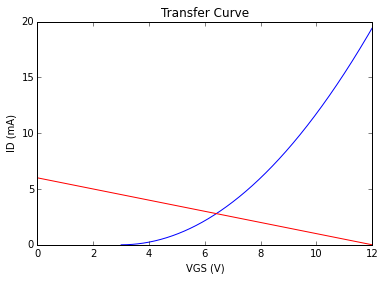## Example 7.7: Page No 367¶

In :
from numpy import arange
from sympy import symbols, solve
%matplotlib inline
from matplotlib.pyplot import plot, title, xlabel, ylabel, show

#IDQ, VDSQ, VGSQ

ID=5e-3# # in amperes
VGS=6# # in volts
VT=3# # in volts
# From Fig. 7.39(a)
VDD=24# # in volts
R1=10# # in mega-ohms
R2=6.8# # in mega-ohms
RD=2.2e3# # in ohms
RS=0.75e3# # in ohms
# Applying Thevnin's theorem to obtain simplified circuit in Fig. 7.39(b)
VGG=VDD*R2/(R1+R2)# # in volts
# Plotting transfer characteristics
k=ID/(VGS-VT)**2# # in amperes per volt square
VGS=arange(3, VGG,0.01)  # Gate source voltage in volts
ID=[]
for vgs in VGS:
ID.append(k*(vgs-VT)**2*1e3) # Drain current in mA ............ (i)

plot(VGS,ID)#
title("Transfer Characteristics")
xlabel("VGS (V)")
ylabel("ID (mA)")
# Plotting bias line
VGS=arange(0, VGG,0.01)# # Gate source voltage in volts
# Writing KVL for the gate-source loop
ID=[]
for x in VGS:
ID.append((VGG-x)/RS*1e3)# # Source current in mA
plot(VGS,ID,"RED")
# Intersection of transfer curve with the bias curve
# Putting VGS = VGG-ID*RD in equation (i) and solving, we get ID**2*RS**2 + (2*RS*VT - 2*VGG*RS - 1/k)*ID + (VGG-VT)**2
# Solving the equation
ID = symbols('ID')
expr = ID**2*RS**2 + (2*RS*VT - 2*VGG*RS - 1/k)*ID + (VGG-VT)**2
IDQ=solve(expr,ID) # in amperes
VGSQ=VGG-IDQ*RS# # in volts
# From the output circuit
VDSQ=VDD-IDQ*(RD+RS)# # in volts
IDQ=IDQ*1e3# # in mili-amperes
print "IDQ = %0.2f mA "%IDQ
print "VDSQ = %0.2f V "%VDSQ
print "VGSQ = %0.2f V "%VGSQ

IDQ = 4.97 mA
VDSQ = 9.35 V
VGSQ = 5.99 V